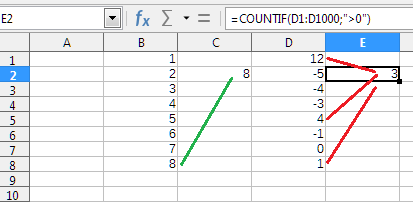# How do I sum the last 12 values in a column?

I’ve been looking at a few related examples but I haven’t quite wrapped my head around how to put it all together.

I have a column B that starts in B2 and will have an ongoing (growing) list of values in rows. I want to SUM (actually, average, but we can just leave it at SUM for the sake of this question and simplicity) the last 12 values regardless of how many rows of data is currently there.

I determined I could use this to determine the row # of the last row with a value:

``````=MAX(NOT(ISBLANK(B2:B10000))*ROW(B2:B10000))-ROW(B2)+2
``````

(Let’s call that “#”) And I imagine some sort of use of the OFFSET function could then be rolled in to start at B# and B(#-12) but I’m unclear how to use a number to reference a row/column number and not a value (I hope I’m explaining that well enough). Also I just learned about OFFSET a few mins ago and I still find its syntax/use a bit confusing. I got as far as determining that this would work for fixed values:

``````=SUM(OFFSET(B2,0,0,12,1))
``````

This is the same as: SUM(B2:B13)

Can someone nudge me the rest of the way home?

If the last cell is B13 or below, than the following formula will work

``````=SUM(OFFSET(INDIRECT("B"&SUMPRODUCT(MAX(IF(B2:B10000="";"";ROW(B2:B10000)))));-12;0;12;1))
``````

Otherwise Err: 502

That does appear to work! Now I need to comb through it so that I understand it and actually learn something.Thanks!

Take your time to study this horror. Surely there are more elegant solutions. It’s just the first thing that came to mind.

In this formula, OFFSET() repels from the very last cell in column B, backs up by 12 cells and shifts down again. If you start from B1, then you can do without INDIRECT()

``````=SUM(OFFSET(B1;SUMPRODUCT(MAX(IF(B2:B10000="";"";ROW(B2:B10000))))-12;0;12;1))
``````

Most likely this can be improved.

The problem here seems to be possibility of empty rows …

``````=SUM(OFFSET(B1;SUMPRODUCT(LARGE(IF(B2:B10000="";"";ROW(B2:B10000));13));0;1000;1))
``````

makes it a bit worse, but allows empty rows (with expectation that last 12 rows would fit into 1000 last rows)…

@mikekaganski, Need to add the IFERROR if there is not at least 12 values.
Why OFFSET only by 1000 rows, and not by 10000?

@LeroyG It seems to me because 1000/12 (months) is over 83 years. If the formula calculates, for example, the average salary for the last year, then 10,000 would probably be too much. It’s hard to imagine an 99-year employee, isn’t it?

By the way, if my guess is correct, then column A should contain dates. And that means all calculations can be tied not to the last filled cell, but to dates.

``=SUMIF(A2:A1000;">"&EOMONTH(MAX(A2:A1000);-13);B2:B1000)``

You can find the last row used in the column by using

``=COUNTIF(B2:B1000,">0")-11``

This can be correct if all values ​​in the column are greater than zero. Otherwise the result will be wrongThe solution could be like this

``=IFERROR(SUM(OFFSET(B2;SUMPRODUCT(MAX(IF(B2:B10000="";"";ROW(B2:B10000))))-13;0;12;1));SUM(B2:B10000))``